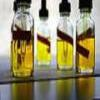#### You may also like### Mathematical Issues for Chemists

A brief outline of the mathematical issues faced by chemistry students.### Reaction Rates

Explore the possibilities for reaction rates versus concentrations with this non-linear differential equation### Catalyse That!

Can you work out how to produce the right amount of chemical in a temperature-dependent reaction?

# Extreme Dissociation

##### Age 16 to 18 Challenge Level:

1 molecule of water dissociated into $H^+$ and $OH^-$ in 100ml of water.
Thus in 1l of water, there will be 10 molecules of $H^+$.

$\therefore [H^+] = 10\ molecules/l$

By dividing by Avogadro's number, this gives:

$[H^+] = 1.66 \times 10^{-23}\ mol/l$

$$\therefore pH = -log_{10}[H^+] = 22.78\ (2\ d.p.)$$

Under the condition that every molecule of water dissociates, we need to make an assumption that 100ml of water weighs approximately 100g.

Therefore 100ml of water is $\frac{100}{18} = 5.56$ moles

Each mole of water gives 1 mole of $[H^+]$.

Therefore $$[H^+] = \frac{5.56}{0.1} = 55.6 mol/l$$

$$\therefore pH = -log_{10}[H^+] = -1.74$$

Under real world situations, the pH of water is 7.

Therefore $[H^+] = 10^{-7} mol/l = 6.02 \times 10^{16} molecules /l$

Therefore there is a single $H^+$ in $\frac{1}{6.02 \times 10^{16}} = 1.66 \times 10^{-17} l$